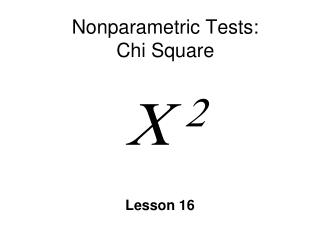DownloadDownload PresentationNonparametric Tests: Chi Square  2

Nonparametric Tests: Chi Square  2

Télécharger la présentationNonparametric Tests: Chi Square  2

- - - - - - - - - - - - - - - - - - - - - - - - - - - E N D - - - - - - - - - - - - - - - - - - - - - - - - - - -
Presentation Transcript

1. Nonparametric Tests:Chi Square 2 Lesson 16

2. Parametric vs. Nonparametric Tests • Parametric hypothesis test • about population parameter (m or s2) • z, t, F tests • interval/ratio data • Nonparametric tests • do not test a specific parameter • nominal & ordinal data • frequency data ~

3. Chi-square (C 2) • Nonparametric tests • same 4 steps as parametric tests • Chi-square test for goodness of fit • single variable • Chi-square test for independence • two variables • Same formula for both • degrees of freedom different • fe calculated differently~

4. Sample Data:2 • Frequency • Expected frequency (fe) • fe = pn • Observed frequency (fo) • S fo = n • Degrees of freedom:Goodness of fit • C-1 • C = number of cells (categories) • C2cvfrom table B.5, page 364 ~

5. Chi-square (C2)

6. Assumptions & Restrictions • Independence of observations • any score may be counted in only 1 category • Size of expected frequencies • If fe < 5 for any cell cannot use C2 • More likely to make Type I error • Solution: use larger sample ~

7. C2 Test for Goodness of Fit • Test about proportions (p) in distribution • 2 different forms of H0 • No preference • category proportions are equal • No difference • from comparison population • e.g., student population • 55% female and 45% male? • H1: the proportions are different ~

8. Female Coke Pepsi Male ½ ½ 55% 45% No preference: H0 No difference: H0 Null Hypotheses: C2

9. SPSS: No Preference • Data in 1 column • Analyze Nonparametric Legacy Dialogs Chi square • Dialogue box • Test Variable List • Expected Values  All categories Equal • Options  Descriptives (frequencies) ~

10. SPSS: No Difference • Same menus as No Preference • But must specify proportions or frequencies • Dialogue box • Expected Values  Values • Specify & Add vales one at time • In same order as defined values for variable in variable view ~

11. *Effect Size: 1 Variable • N = total sample size across all categories • df = #categories – 1 • zero = no difference • 1 = large difference ~

12. C2 Test for Independence • 2 variables • are they related or independent • H0: • distribution of 1 variable is the same for the categories of other • no difference • Same formula as Goodness of Fit • different df ~

13. C2 Test for Independence • Differences from Goodness of Fit • df = (R-1)(C-1) • R = rows • C = columns • Expected frequency for each cell

14. Example • Does watching violent TV programs cause children to be more aggressive on the playground? • Data: frequency data • Violent program: yes or no • Aggressive: yes or no ~

15. 9 41 17 33 C2 Test for Independence Aggressive Yes No Violent TV Yes No

16. SPSS: Test for Independence • Two variables • Two-Way Contingency Table Analysis • Data: 1 column for each variable • Analyze Descriptives Crosstabs • Dialogue Box • Variables  Rows or Columns • Statistics  Chi Square, *Phi ~

17. Effect Size (): 2 Variables • N = total sample size across all categories • Phi values: 0-1 • Interpret similar to Pearson’s r • Small = .1; medium = .3, large = .5 ~